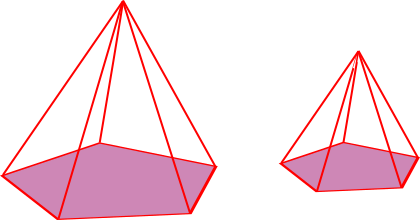# Exam-Style Questions.

## Problems adapted from questions set for previous Mathematics exams.

### 1.

GCSE Higher

The circumference of the red circle is 80% of the circumference of the blue circle.

(a) Find the ratio of the area of the red circle to the area of the blue circle.

The green square has sides of length $$a$$ cm.

The yellow square has sides of length $$b$$ cm.

The area of the green square is 21% greater than the area of the yellow square.

(b) Work out the ratio $$a : b$$

### 2.

GCSE Higher

The three boxes pictured below are mathematically similar.

The edges of the largest box are twice as long as the edges of the smallest box.

The volume of the middle box is twice as big as the volume of the smallest box.

What fraction is the volume of the middle box of the volume of the largest box?### 3.

GCSE Higher

Two similar pentagonal based pyramids have surface areas 200 cm2 and 50 cm2 respectively.The volume of the larger pyramid is 800 cm3.

Show that the volume of the smaller pyramid is 100 cm3.

If you would like space on the right of the question to write out the solution try this Thinning Feature. It will collapse the text into the left half of your screen but large diagrams will remain unchanged.

The exam-style questions appearing on this site are based on those set in previous examinations (or sample assessment papers for future examinations) by the major examination boards. The wording, diagrams and figures used in these questions have been changed from the originals so that students can have fresh, relevant problem solving practice even if they have previously worked through the related exam paper.

The solutions to the questions on this website are only available to those who have a Transum Subscription.

Exam-Style Questions Main Page

Search for exam-style questions containing a particular word or phrase:

To search the entire Transum website use the search box in the grey area below.